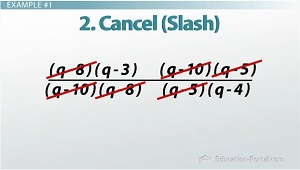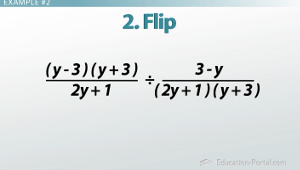# Multiplying and Dividing Rational Expressions: Practice Problems

An error occurred trying to load this video.

Try refreshing the page, or contact customer support.

Coming up next: How to Add and Subtract Rational Expressions

### You're on a roll. Keep up the good work!

Replay
Your next lesson will play in 10 seconds
• 0:05 Review
• 0:24 Example #1
• 1:44 Example #2
• 3:14 Example #3
• 4:16 Lesson Summary
Save Save

Want to watch this again later?

Timeline
Autoplay
Autoplay
Speed Speed

#### Recommended Lessons and Courses for You

Lesson Transcript
Instructor: Kathryn Maloney

Kathryn teaches college math. She holds a master's degree in Learning and Technology.

Let's continue looking at multiplying and dividing rational polynomials. In this lesson, we will look at a couple longer problems, while giving you some practice multiplying and dividing.

## Review

Multiplication and division of rational polynomial expressions is easy once you remember the steps.

For multiplication: factor, cancel or slash, and multiply.

For division: factor, flip, cancel or slash, and multiply.

Let's do some larger problems.

## Example #1((q^2 - 11q + 24) / (q^2 - 18q + 80)) * ((q^2 - 15q + 50) / (q^2 - 9q + 20))

First, we need to factor. (q^2 - 11q + 24) factors into (q - 8)(q - 3). (q^2 - 18q + 80) factors into (q - 10)(q - 8). (q^2 - 15q + 50) factors into (q - 10)(q - 5). (q^2 - 9q + 20) factors into (q - 5)(q - 4).

So, this is what our new expression is going to look like: ((q - 8)(q - 3) / (q - 10)(q - 8)) * ((q - 10)(q - 5) / (q - 5)(q - 4))

Next, we are going to cancel (what I like to call slash) like terms. We're going to cancel or slash (q - 10) over (q - 10), (q - 8) over (q - 8), and finally (q - 5) over (q - 5).

Now that we have canceled or slashed all of the like terms from the top and bottom, we multiply straight across. Don't multiply anything we slashed because those are now 1's. It turns out, our answer is (q - 3) / (q - 4).

## Example #2((y^2 - 9) / (2y + 1)) / ((3 - y) / (2y^2 + 7y + 3))

Let's factor. (y - 9) = (y - 3)(y + 3) and (2y^2 + 7y + 3) = (2y + 1)(y + 3). Our next step is to flip the second fraction and change it to multiplication. Our new expression is going to look like this: ((y - 3)(y + 3) / (2y + 1)) * ((2y + 1)(y + 3) / ((3 - y)).

To unlock this lesson you must be a Study.com Member.

### Register to view this lesson

Are you a student or a teacher?

#### See for yourself why 30 million people use Study.com

##### Become a Study.com member and start learning now.
Back
What teachers are saying about Study.com

### Earning College Credit

Did you know… We have over 200 college courses that prepare you to earn credit by exam that is accepted by over 1,500 colleges and universities. You can test out of the first two years of college and save thousands off your degree. Anyone can earn credit-by-exam regardless of age or education level.